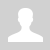Binary tree

## Data structure. Binary tree

Concept of a tree

Trees are a very common data structure in many areas of computer science and other contexts. A family tree is an example of a tree, and a folder structure where a root directory has many folders and sub-folders is another example. Like a tree in nature, a rooted tree has a root, branches and leaves, the difference being that a rooted tree in computer science has its root at the top and its leaves at the bottom.

Typical uses for rooted trees include:

• Manipulating hierarchical data, such as folder structures or moves in a game
• Making information easy to search (see binary tree search below)
• Manipulating sorted lists of data

Generations of a family may be thought of as having a tree structure: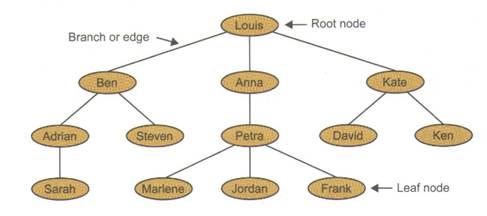The tree shown above has a root node, and is therefore defined as a rooted tree. Here are some terms used in connection with rooted trees:

Node: the nodes contain the tree data.

Edge: an edge connects two nodes. Every node except the root is connected by exactly one edge from another node in the level above it.

Root: this is the only node that has no incoming edges.

Child: The set of nodes that have incoming edges from the same node.

Parent: a node is a parent of all the nodes it connects to with outgoing edges.

Subtree: the set of nodes and edges comprised of a parent and all descendants of the parent. A subtree may also be a leaf.

Leaf node: a node that has no children.

Q1: Identify the left subtree of the root, the parent of Frank and the children of Kate. How many parent nodes are there in the tree? How many child nodes?

Note that a rooted tree is a special case of a connected graph. A node can only be connected to one parent node, and to its children. It is described as having has no cycles because there can be no connection between children, or between branches, for example form Ben to Anna or Petra to Kate.

A more general definition of a tree

A tree is a connected, undirected graph with no cycles. “Connected” implies that it is always possible to find a path from a node to any other node, by backtracking if necessary. “No cycles” means that it is not possible to find a path in the tree which returns to the start node without traversing an edge twice. Note that a tree does not have to have a root.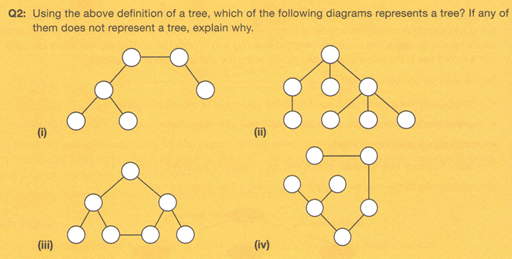A binary search tree

A binary tree is a rooted tree in which each node has a maximum of two children. A binary search tree holds items in such a way that a tree can be searched quickly and easily added, and the whole tree can be printed out in sequence.

17, 8, 4, 12, 22, 19, 14, 5, 30, 25

The tree is constructed using the following algorithm:

Place the first item at the root. Then for each item in the list, visit the root, which becomes the current node, and the branch left f the item is less than the value at the current node, and the right if the item is greater that the value at the current node. Continue down the branch, applying the rule at the each node visited, until a leaf node is reached. The item is then placed to the left or right on this node, depending on whether it is less than or greater than the value at the node.

Following this algorithm, 17 is placed at the root, 8 is less than 17, so it is placed at a new node to the left of the root. 4 is less than 17, so we branch left at the root, branch left at 8, and place it to the left. 12 is less than 17, so we branch left at the root, branch right at 8, and place it to the right. The final tree looks like this: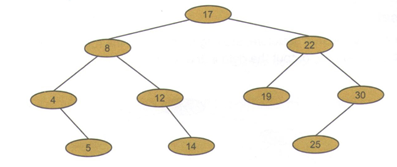To search the tree for the number 19, for example, we follow the same steps.

19 is greater than 17, so branch right.

19 is than 17, so branch left. There it is!

Q3:

1. Which nodes will be visited when searching for the number 14?
2. Which nodes will be visited when searching for the number 21, which is not in the tree?

(c)Where will new nodes 10 and 20 be inserted?

Traversing a binary tree

There are three ways of traversing a tree:

• Pre-order traversal
• In-order traversal
• Post-order traversal

The name refer to whether the root of each sub-tree is visited before, between of after both branches have been traversed.

Pre-order traversal

Draw an outline around the tree structure, starting to the left of the root. As you pass to the left of a node (where the red dot is marked), output the data in that node.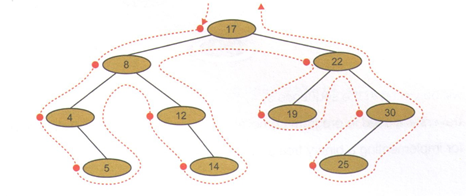The nodes will be visited in the sequence 17, 8, 4, 5, 12, 14, 22, 19, 30 ,25

A pre-order traversal may be used to produce prefix notation, used in functional programming languages. A simple illustration would be a function statement, x = sum a, b rather than x = a + b, in which the operation comes before the operations rather than between them, as in infix notation.

In-order traversal

Draw an outline around the tree structure, starting to the left of the root. As you pass underneath a node (where the red dot is marked), output the data in that node.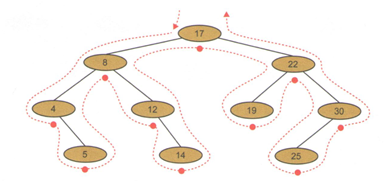The nodes will be visited in the sequence 4, 5, 8, 12, 14, 17, 19, 22, 25, 30

The in-order traversal visits the nodes in sequential order.

Q4: Construct a binary search tree to hold the names Mark, Stephanie, Paul, Anne, Hanna, Luke, David, Vincent, Tom. List the names, in the order they would be checked, to find David.

Q5: List the names in the order they would be output when an in-order traversal is performed.

Post-order traversal

Draw an outline around the tree structure, starting to the left of the root. As you pass to the right of a node (where the red dot is marked), output the data in that node.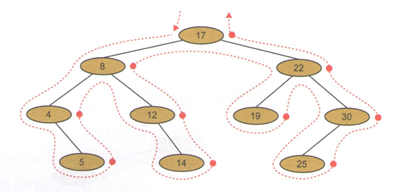The nodes will be visited in the sequence 5, 4, 14, 12, 8, 19, 25, 30 ,22, 17

Post-order traversal is used in program compilation to produce Reverse Polish Notation (Chapter55).

Algorithms for implementing a binary tree and each of these traversals will be covered in chapter 44.

Implementation of a binary search tree

• A binary search tree can be implemented using an array of records, with each node consisting of:
• Left pointer
• Data item
• Right pointer

Alternatively, it could be held in a list of tuples, or three separate lists of array, one for each of the pointers and one for the data items.

The numbers 17, 8, 4, 12, 22, 19, 14, 5, 30, 25 used to construct the tree above could be held as follows:

 left data right Tree  1 17 4 Tree  2 8 3 Tree  -1 4 7 Tree  -1 12 6 Tree  5 22 8 Tree  -1 19 -1 Tree  -1 14 -1 Tree  -1 5 -1 Tree  9 30 -1 Tree  -1 25 -1

For an example, the left pointer in tree  points to tree  and the right pointer points to tree . The value -1 is a ‘rogue value’ which indicates that there is no child on the relevant side (left or right).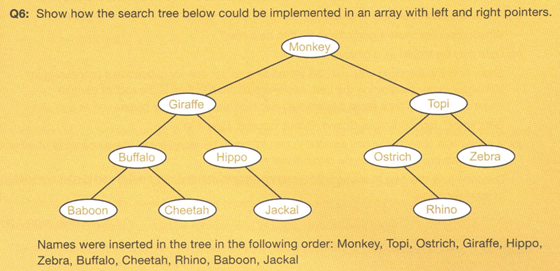Источник: https://www.amazon.com/AQA-AS-Level-Computer-Science/dp/1910523070/ref=sr_1_19?dchild=1&keywords=computer+science+a-leve
Категория: Data structures | Добавил: bzfar77 (23.06.2020)
Просмотров: 167 | | Рейтинг: 5.0/2
 Всего комментариев: 0